output.to from Sideway
Algebra

Draft for Information Only

# Content

`  Binomial Theorem and Binomial Series[]   Binomial Expansion[]   Pascal's Triangle[]    Properties of Pascal's Triangle   Binomial Theorem[]   Binomial Series[]`

## Binomial Theorem and Binomial Series[]

A binomial is an algebraic polynomial expression of form (a+b). The binomial theorem is a theorem descibing the algebraic expansion of the powers of a binomial into an infinite binomial series.

### Binomial Expansion[]

The expansion of the powers of a binomial can be obtained by direct multiplication. Imply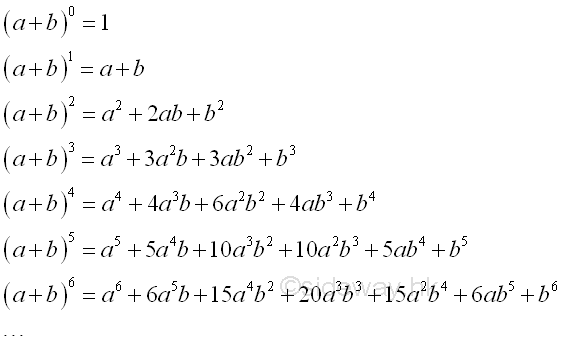The number of terms of the binomial expansion is alway equal to the power of the binomial plus 1. The exponent of a decreases by 1 from the power of the binomial while the exponent of b increases by 1 from zero or vice versa. Therefore the first term is a to the power of the binomial and the last term is b to the power of the binomial, and the sum of the exponents of a and b is always equal to the power of the binomial. The coefficient of a is a specific integer depending on the power of the binomial and the exponent of a or b or vice versa. The coefficient of each term can be obtained by multiplying the coefficient of previous term by the exponent of a in the previous term and dividing the product by the item number of the previous term instead of direct multiplaction.

### Pascal's Triangle[]

The coefficients of powers of binomial, (a+b) can be arranged in the format of Pascal triangle with the first coefficient of the powers of binomial on the same row or column.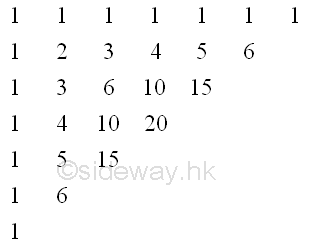The coefficients of powers of binomial can also be arranged in the an "equilateral" triangle pattern of same numbers of coefficients on the edge. Imply

 0 1 1 1 1 2 1 2 1 3 1 3 3 1 4 1 4 6 4 1 5 1 5 10 10 5 1 6 1 6 15 20 15 6 1 7 1 7 21 35 35 21 7 1 8 1 8 28 56 70 56 28 8 1 9 1 9 36 84 126 126 84 36 9 1 10 1 10 45 120 210 252 210 120 45 10 1 11 1 11 55 165 330 462 462 330 165 55 11 1 12 1 12 66 220 495 792 924 792 495 220 66 12 1 13 1 13 78 286 715 1287 1716 1716 1287 715 286 78 13 1 14 1 14 91 364 1001 2002 3003 3432 3003 2002 1001 364 91 14 1 15 1 15 105 455 1365 3003 5005 6435 6435 5005 3003 1365 455 105 15 1

The row number on the left is equal to the power of the binomial with the expanded binomial on the left. Similarly, the exponent of a decreases by 1 from the power of the binomial to zero while the exponent of b increases by 1 from zero to the power of the binomial or vice versa. The number of term in each row is equal to the power of the binomial plus. And the sum of the coefficient of the binomial expansion in each row is equal to 2 to the power of the power of the binomial. With this rearrangement, the coefficient of each term is equal to the sum of the two coefficients of the row above immediately. The coefficients of powers of binomial can therefore be determined by the pattern of a Pascal's triangle, rather than direct multiplication.

#### Properties of Pascal's Triangle

• The sum of the numbers in any row is equal to 2 to the nth power or 2n, when n is the number of the row. e.g. 24 = 1+4+6+4+1 = 16

• If the 2nd number in a row is a prime number, all the numbers in that row excluding the number 1 are divisible by the number. e.g "1 7 21 35 35 21 7 1" of row 7 are all divisible by 7 except 1.

•  The sum of the numbers of any length, i, in any diagonal is equal to the next number in the cross diagonal of a hockey stick like pattern. e.g. 1+5+15+35=56 or 1+4+10=15

• The cummulative sum of the numbers of any length, i, in a diagonal from first diagonal to is equal to the next number in next diagonal of a parallelogram pattern. e.g. (1+1+1+1+1)+(1+2+3+4+5)=21 the 6th number of third diagonal.

• The first diagonal is  a sequence of 1s.

• The second diagonal is a sequence of counting numbers, 1,2,3,4.

• The third diagonal is  a sequence of triangular numbers, 1,3,6,10....

• The fourth diagonal is a sequence of tetrahedral numbers, 1,4,10,20,35....

• The fifth diagonal is a sequence of pentatope numbers, 1,5,15,35,70,....

• The sequence of numbers after the addition of the next number to the previous number in the third diagonal is a sequence of square numbers, 1, 1+3,3+6,6+10,....

• The numbers in any row, i, is a magic 11's and is equal to 11 to the ith power by considering each number is arranged in decimal dight order. e.g. 118=214358881=1  8  28  56  70  56  28  8 1

• The sequence of numbers after the consecutive addition of the  next number of the previous row to the number in the first diagonal is a Fibonnacci's sequence, 1,  1,  1+1, 1+2, 1+3+1, 1+4+3, 1+5+6+1, 1+6+10+4.

• The numbers in any row is the numbers of figures formed by all possible lines connected by points on a circle. e.g. 7th row: 1 circle, 7 points, 21 segments, 35 triangles, 35 quadrilaterals, 21 pentagons, 7 hexagons, 1 heptagons.

•  By Harian Brothers, constant e can be calculated from the product  of consecutive multiplication of all numbers in a row and is obtained by the product of last row n+1 times the product of third last row n-1 and divided by the square of the product of the second last row when  taking limit as n approaching infinity.

• The Star of David Theorems. The product of a number in a row, the second next number in next row and the next number in second next row is equal to the product of the next number in the same row, the previous number in the next row and the next number in the third row. The greatest common divisors of these two groups of numbers are the equal

• Catalan Numbers

• Sums of the Binomial Reciprocals

• Squares

• Squares of the Binomials

• Cubes

• pi in Pascal

• Sierpinski's Triangle

### Binomial Theorem[]

The binomial theorem is developed to expand binomials of any given power directly by describing the algebraic expression of the general form of a binomial expansion. The binomial theorem states that the binomial (a+x) to the power of a nonnegative integer n can be expressed as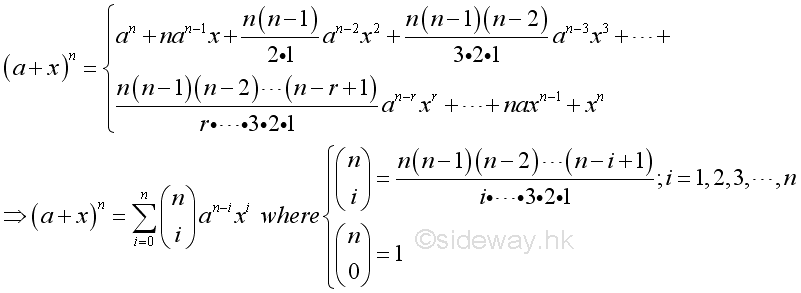If a=1, then the general binomial series can be reduced to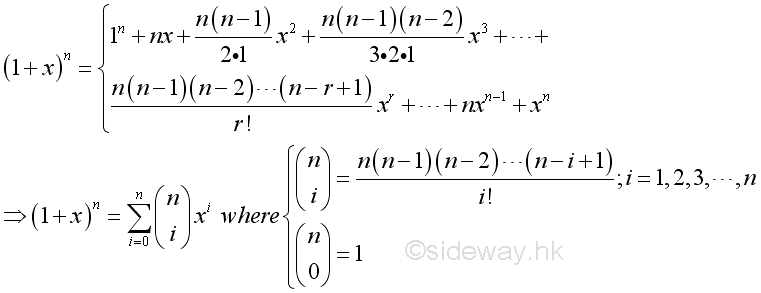### Binomial Series[]

In fact, the number n in the binomial theorem can be extended to any number. For any number n, the expansion of the binomial should be expressed in form of an infinite series, called binomial series.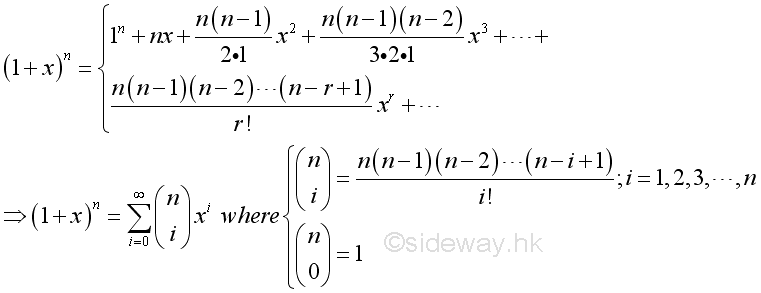For any number n, if the absolute value of x is less than one, then the series converges absolutely.

If n is a nonnegative integer, the infinite binomial series is reduced to a finite series having the same form of the binomial theorem

©sidewayReferences

1. B. Joseph, 1978, University Mathematics: A Textbook for Students of Science & Engineering, Blackie & Son Limited, HongKong
2. Wheatstone, C., 1854, On the Formation of Powers from Arithmetical Progressions, Proceedings of The Royal Society of London, Vol 7, p145-151,, London
3. Stroud, K.A., 2001, Engineering Mathematics, Industrial Press, Inc, NY
4. Coolidge, J.L., 1949, The Story of The Binomial Theorem, The American Mathematical Monthly, Vol 56, No.3, Mar, pp147-157ID: 130500022 Last Updated: 2016/3/22 Revision: 1 Ref:Home (5)

Business

Management

HBR (3)

Information

Recreation

Hobbies (7)

Culture

Chinese (1097)

English (336)

Reference (66)

Computer

Hardware (149)

Software

Application (187)

Digitization (24)

Numeric (19)

Programming

Web (644)CSS (SC)

ASP.NET (SC)

HTML

Knowledge Base

Common Color (SC)

Html 401 Special (SC)

OS (389)MS Windows

Windows10 (SC)

.NET Framework (SC)

DeskTop (7)

Knowledge

Mathematics

Formulas (8)

Number Theory (206)

Algebra (20)

Trigonometry (18)

Geometry (18)

Calculus (67)

Complex Analysis (21)

Engineering

Tables (8)

Mechanical

Mechanics (1)

Rigid Bodies

Statics (92)

Dynamics (37)

Fluid (5)

Control

Acoustics (19)

Biology (1)

Geography (1)

Latest Updated Links

Copyright © 2000-2019 Sideway . All rights reserved Disclaimers last modified on 10 Feb 2019